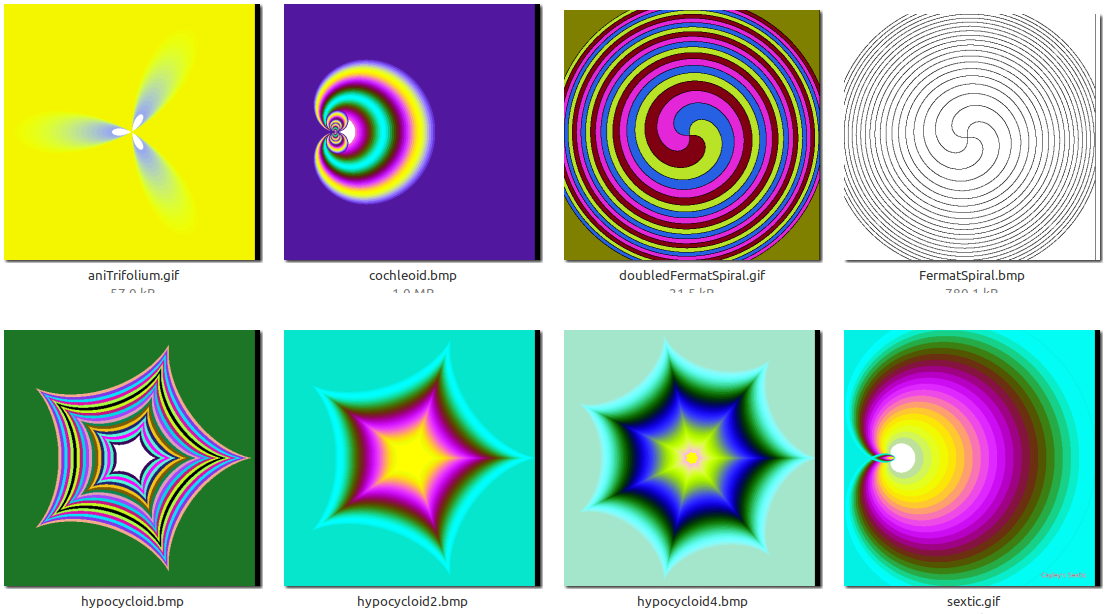# Families of curves, colour filled

A favourite coding activity for me is generating unusual mathematical figures and playing with the parameters. Many have traditional names, and I'd just found a reference page with several that were new to me.Curves to display in 2D are usually in Cartesian ( x, y) coordinates, or polar ( r, theta). I've variations on the following code for generating coloured families of trochoids, cycloids, cochleoid, trifoliuM, sextic, Fermat spiral, etc. Using colour sequential fills and adding progressive rotations produces eye-catching graphics.## Code

```
'  ratchet curve

nomainwin

pi      =4 *atn( 1)

Cx  =251    '   centre to fill from
Cy  =251
r   = 30    '   Initial colour values for the fill.
g   =260
bl  = 20

WindowWidth  =500
WindowHeight =550

open "Ratchet pawl" for graphics_nsb as #wg

hw      =hwnd( #wg)
calldll #user32, "GetDC", hw as ulong, hdc as ulong

#wg "trapclose quit"

#wg "home"

state\$ ="up"

for a =140 to 10 step -2

re          =int( 128 +128 *cos( a /7))
gr          =int( 128 +128 *sin( a /19))
bl          =int( 128 +128 *cos( a /37))

fillCol\$    =str\$( re)     +" " +str\$( gr)   +" "   +str\$( bl)

#wg "color ";     fillCol\$
#wg "backcolor "; fillCol\$

targetcolor   =bl *2^16    +gr *2^8    +re      '   this is colour to fill WITH
'   this is also the colour of the outline to fill out TO.

for theta =0 to 2 *pi +0.002 step 0.001
scan
r   =  a +int( ( ( 1.5 *( theta)) mod pi) *( a /5))
x   =250 +r *cos( theta +a /130)
y   =250 -r *sin( theta +a /130)
if state\$ ="down" then
#wg "goto "; int( x); " "; int( y)
else
#wg "goto "; int( x); " "; int( y)
#wg "down"
state\$ ="down"
end if
next theta

'goto [skip]
calldll #gdi32, "ExtFloodFill",_
hdc                as ulong,_
Cx                 as  long,_
Cy                 as  long,_
targetcolor        as  long,_                '   ie fill out 'til this colour is met..
_FLOODFILLBORDER   as  long,_
result             as  long
[skip]
next a

#wg "getbmp scr 0 0 500 500"
bmpsave "scr", "ratchet6d.bmp"

wait

sub quit h\$
calldll #user32, "ReleaseDC", hw as ulong, hdc as ulong, ret as void   'release the DC
close #wg
end
end sub
```